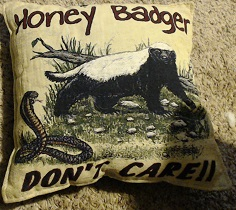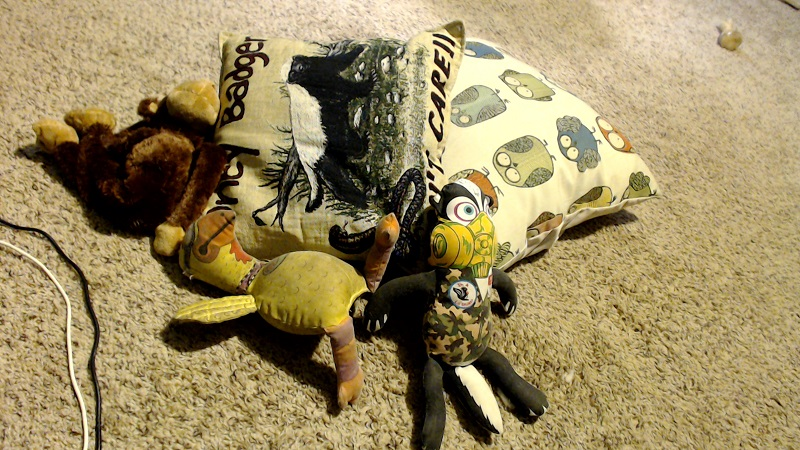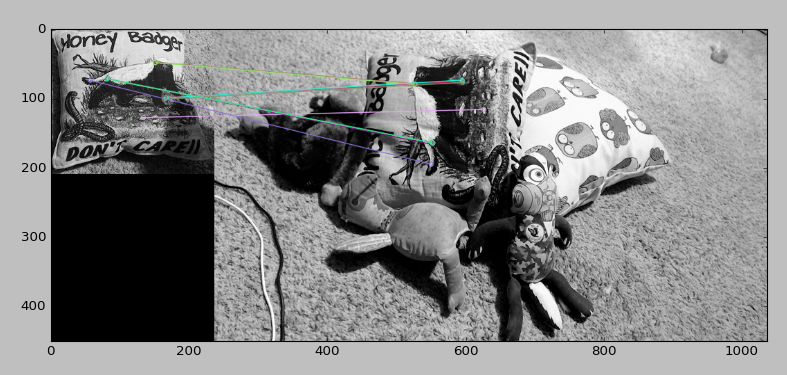## Feature Matching (Homography) Brute Force OpenCV Python Tutorial

Welcome to a feature matching tutorial with OpenCV and Python. Feature matching is going to be a slightly more impressive version of template matching, where a perfect, or very close to perfect, match is required.

We start with the image that we're hoping to find, and then we can search for this image within another image. The beauty here is that the image does not need to be the same lighting, angle, rotation...etc. The features just need to match up.

To start, we need some sample images. Our "template," or image we're going to try to match:Then our image to search for this template in:Here, our template image is a bit smaller in the template than in the image we're going to search. It is also a different rotation, and has some different shadows.

Now we're going to use a form of "brute force" matching. We're going to find all features in both images. Then we match these features. We then can draw out as many as we want. Careful though. If you draw say 500 matches, you're going to have a lot of false positives. Draw the first few only.

```import numpy as np
import cv2
import matplotlib.pyplot as plt

So far we've imported the modules we're going to use, and defined our two images, the template (img1) and the image we're going to search for the template in (img2).

`orb = cv2.ORB_create()`

This is the detector we're going to use for the features.

```kp1, des1 = orb.detectAndCompute(img1,None)
kp2, des2 = orb.detectAndCompute(img2,None)```

Here, we find the key points and their descriptors with the orb detector.

`bf = cv2.BFMatcher(cv2.NORM_HAMMING, crossCheck=True)`

This is our BFMatcher object.

```matches = bf.match(des1,des2)
matches = sorted(matches, key = lambda x:x.distance)```

Here we create matches of the descriptors, then we sort them based on their distances.

```img3 = cv2.drawMatches(img1,kp1,img2,kp2,matches[:10],None, flags=2)
plt.imshow(img3)
plt.show()```

Here, we've drawn the first 10 matches. The output:The next tutorial:• Drawing and Writing on Image OpenCV Python Tutorial

• Image Operations OpenCV Python Tutorial

• Image arithmetics and Logic OpenCV Python Tutorial

• Thresholding OpenCV Python Tutorial

• Color Filtering OpenCV Python Tutorial

• Blurring and Smoothing OpenCV Python Tutorial

• Morphological Transformations OpenCV Python Tutorial

• Canny Edge Detection and Gradients OpenCV Python Tutorial

• Template Matching OpenCV Python Tutorial

• GrabCut Foreground Extraction OpenCV Python Tutorial

• Corner Detection OpenCV Python Tutorial

• Feature Matching (Homography) Brute Force OpenCV Python Tutorial
• MOG Background Reduction OpenCV Python Tutorial

• Haar Cascade Object Detection Face & Eye OpenCV Python Tutorial